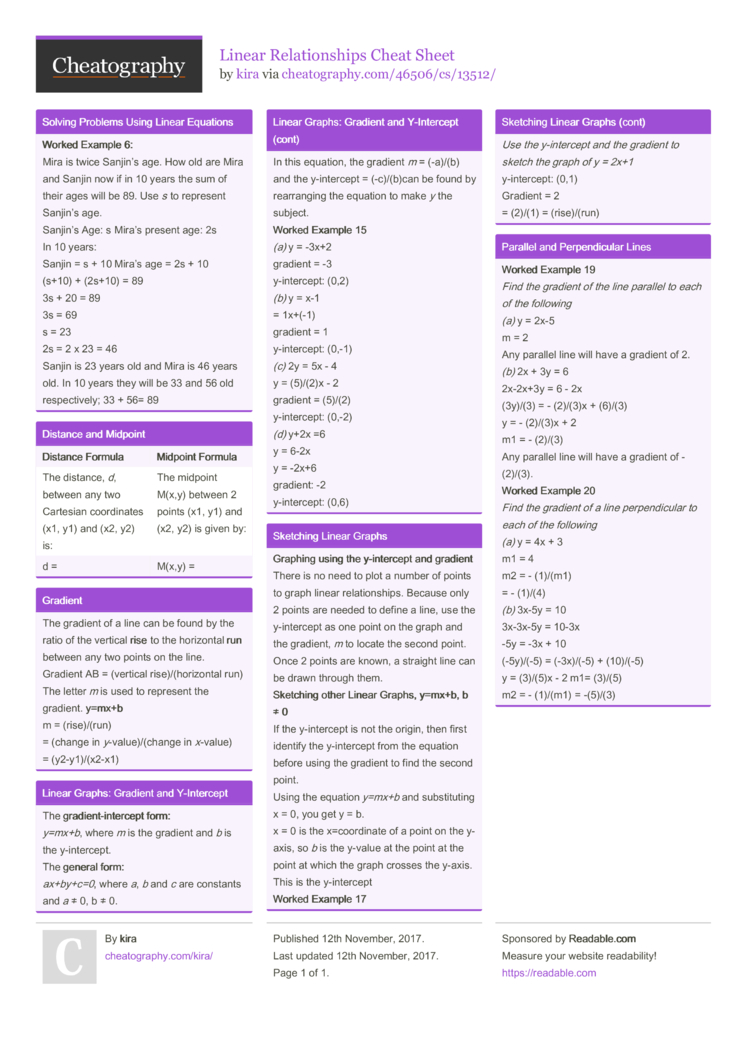# Linear Relationships Cheat Sheet by kira

### Solving Problems Using Linear Equations

 Worked Example 6: Mira is twice Sanjin’s age. How old are Mira and Sanjin now if in 10 years the sum of their ages will be 89. Use s to represent Sanjin’s age. Sanjin’s Age: s Mira’s present age: 2s In 10 years: Sanjin = s + 10 Mira’s age = 2s + 10 (s+10) + (2s+10) = 89 3s + 20 = 89 3s = 69 s = 23 2s = 2 x 23 = 46 Sanjin is 23 years old and Mira is 46 years old. In 10 years they will be 33 and 56 old respec­tively; 33 + 56= 89

### Distance and Midpoint

 Distance Formula Midpoint Formula The distance, d, between any two Cartesian coordi­nates (x1, y1) and (x2, y2) is: The midpoint M(x,y) between 2 points (x1, y1) and (x2, y2) is given by: d = M(x,y) =

 The gradient of a line can be found by the ratio of the vertical rise to the horizontal run between any two points on the line. Gradient AB = (vertical rise)/­(ho­riz­ontal run) The letter m is used to represent the gradient. y=mx+b m = (rise)­/(run) = (change in y-va­lue­)/(­change in x-value) = (y2-y1­)/(­x2-x1)

### Linear Graphs: Gradient and Y-Inte­rcept

 The grad­ien­t-i­nte­rcept form: y=mx+b, where m is the gradient and b is the y-inte­rcept. The general form: ax+by­+c=0, where a, b and c are constants and a ≠ 0, b ≠ 0. In this equation, the gradient m = (-a)/(b) and the y-inte­rcept = (-c)/(­b)can be found by rearra­nging the equation to make y the subject. Worked Example 15 (a) y = -3x+2 gradient = -3 y-inte­rcept: (0,2) (b) y = x-1 = 1x+(-1) gradient = 1 y-inte­rcept: (0,-1) (c) 2y = 5x - 4 y = (5)/(2)x - 2 gradient = (5)/(2) y-inte­rcept: (0,-2) (d) y+2x =6 y = 6-2x y = -2x+6 gradient: -2 y-inte­rcept: (0,6)

### Sketching Linear Graphs

 Graphing using the y-inte­rcept and gradient There is no need to plot a number of points to graph linear relati­ons­hips. Because only 2 points are needed to define a line, use the y-inte­rcept as one point on the graph and the gradient, m to locate the second point. Once 2 points are known, a straight line can be drawn through them. Sket­ching other Linear Graphs, y=mx+b, b ≠ 0 If the y-inte­rcept is not the origin, then first identify the y-inte­rcept from the equation before using the gradient to find the second point. Using the equation y=mx+b and substi­tuting x = 0, you get y = b. x = 0 is the x=coor­dinate of a point on the y-axis, so b is the y-value at the point at the point at which the graph crosses the y-axis. This is the y-inte­rcept Worked Example 17 Use the y-inte­rcept and the gradient to sketch the graph of y = 2x+1 y-inte­rcept: (0,1) Gradient = 2 = (2)/(1) = (rise)­/(run)

### Parallel and Perpen­dicular Lines

 Worked Example 19 Find the gradient of the line parallel to each of the following (a) y = 2x-5 m = 2 Any parallel line will have a gradient of 2. (b) 2x + 3y = 6 2x-2x+3y = 6 - 2x (3y)/(3) = - (2)/(3)x + (6)/(3) y = - (2)/(3)x + 2 m1 = - (2)/(3) Any parallel line will have a gradient of - (2)/(3). Worked Example 20 Find the gradient of a line perpen­dicular to each of the following (a) y = 4x + 3 m1 = 4 m2 = - (1)/(m1) = - (1)/(4) (b) 3x-5y = 10 3x-3x-5y = 10-3x -5y = -3x + 10 (-5y)/(-5) = (-3x)/(-5) + (10)/(-5) y = (3)/(5)x - 2 m1= (3)/(5) m2 = - (1)/(m1) = -(5)/(3)

## Download the Linear Relationships Cheat Sheet1 Page
//media.cheatography.com/storage/thumb/kira_linear-relationships.750.jpg

PDF (recommended)